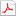검색어 입력폼

# [전기전자전파] 물리전자공학 1장 연습문제풀이

저작시기 2009.04 |등록일 2009.04.21어도비 PDF (pdf) | 11페이지 | 가격 2,000원

물리전자공학 1장 연습문제풀이

없음

## 본문내용

SOLUTIONS CHAPTER 1
1.2. Consider a lithium nucleus, of charge +3q. Calculate the first three electron energies for an electron in a Li++ ion, using the Bohr model.

1.3. In each of the potential energy distributions in Figure 1P.1, sketch the magnitude and direction of the force on the electron.

1.5. Consider the electron in the energy diagram of Figure 1P.2. Taking the energy the electron has at Point A as Etotal, at each of the indicated positions, find the total energy, the kinetic energy, the potential energy, and the electron’s velocity. Indicate the direction of force (if any). Recall that total energy is conserved. At point “D” the electron collides inelastically with something (perhaps an atom in the crystal). After the collision, the electron’s energy is equal to its potential energy, and its kinetic energy is zero. Its total energy is much less than before the collision; where did the extra energy go?

없음